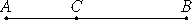# Proposition 77

If from a straight line there is subtracted a straight line which is incommensurable in square with the whole, and which with the whole makes the sum of the squares on them medial but twice the rectangle contained by them rational, then the remainder is irrational; let it be called that which produces with a rational area a medial whole.

From the straight line AB let there be subtracted the straight line BC which is incommensurable in square with AB and fulfills the given conditions.

I say that the remainder AC is the irrational straight line aforesaid.Since the sum of the squares on AB and BC is medial, while twice the rectangle AB by BC is rational, therefore the sum of the squares on AB and BC is incommensurable with twice the rectangle AB by BC. Therefore the remainder, the square on AC, is also incommensurable with twice the rectangle AB by BC.

And twice the rectangle AB by BC is rational, therefore the square on AC is irrational. Therefore AC is irrational. Let it be called that which produces with a rational area a medial whole.

Q.E.D.

## Guide

This proposition is used for a few later propositions in Book X starting with X.83.